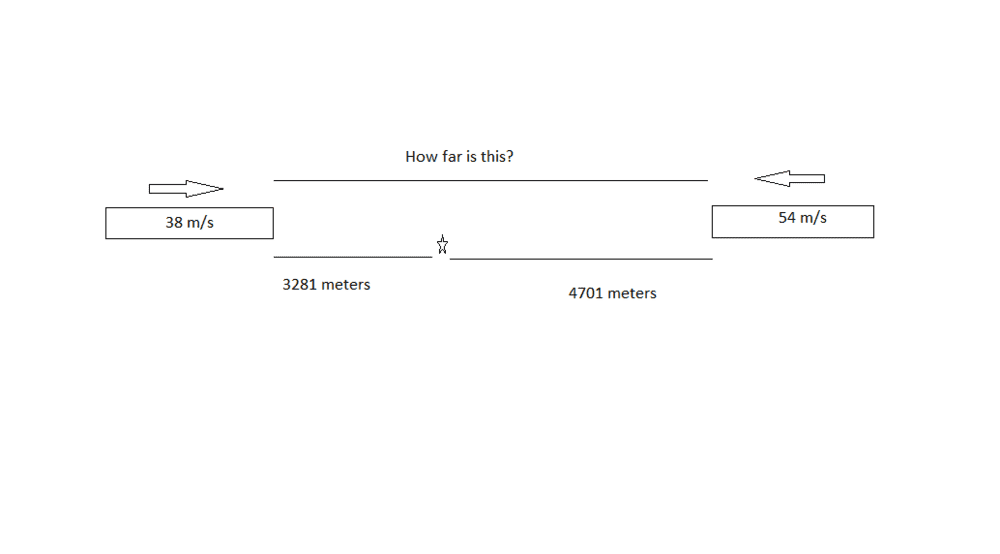Train deceleration and minimum stopping distances

Homework Statement:
Two trains are traveling on the same linear track but in opposite direction and toward each other. The train on the right is moving to the left with a velocity of 54 m/s and the train on the left is moving to the right with a velocity of 38 m/s. When the brakes are fully applied on the train on the right, the ensuing acceleration has a magnitude of 0.31 m/s2.

Given the trains have their breaks applied simultaneously and come to a stop at exactly the same instant,
--determine the minimum distance between the trains at which they could fully apply their brakes and come to a complete halt without colliding.
--determine the acceleration of the train on the left.
Relevant Equations:
x= 1/2at^2+Vot+Xo
a= delta v/t
So my work includes using the acceleration formula a=delta v/t

(Vrtf-Vrti)/a -> (0-54)/(-0.31) -> t=174 seconds

I plug in 174 seconds to find the acceleration of the left train. and got -0.22m/s^2

I then used the displacement equation
x=(1/2)at^2+Vo+So
coming out with Xrt=4703m and Xlt= 3281m

I subtracted those coming out with 1422m, which is the minimum distance between each train to safely halt without crashing.

is that right?!

hmmm27
Gold Member
The answer I came up with for ##distance_{initial}## (through a slightly different route that didn't include calculating the time because it isn't required) is the same as the answer you'll come up with if you doublecheck your signage.

•Delta2
what was the route you took?

haruspex
Homework Helper
Gold Member
I subtracted those
Umm... why?

Umm... why?

it seemed like the good option... I'm guess that wasn't the right pathway :/

PeroK
Homework Helper
Gold Member
2021 Award
it seemed like the good option... I'm guess that wasn't the right pathway :/
If the first train takes about ##4.7km## to stop and the second train takes about ##3.3 km## to stop, then what happens if they start about ##1.4 km## apart?

hmmm27
Gold Member
While we're at it, in part one, is "##-##" the correct notation for the direction of the velocity ? given the wording of the question.

jbriggs444